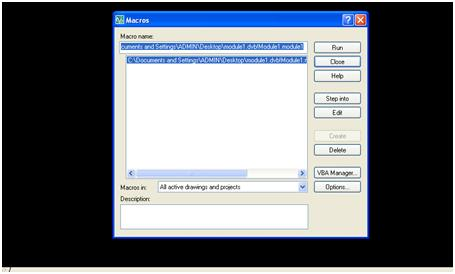• 1 Vote(s) - 4 Average
• 1
• 2
• 3
• 4
• 5
 Example 1 : Draw a line using AutoCAD VBAtechberthModeratorPosts: 55 Threads: 55 Joined: Nov 2019 Reputation: 18 21-11-2019, 05:41 AM (This post was last modified: 21-11-2019, 05:44 AM by techberth.) Example 1 : Draw a line using AutoCAD VBA 'Set Start point and End Point Variables Dim Startpoint(0 To 2) As Double Dim Endpoint(0 To 2) As Double 'Start Coordinates XYZ values Startpoint(0) = 100: Startpoint(1) = 100: Startpoint(2) = 0 'End Coordinates XYZ values Endpoint(0) = 200: Endpoint(1) = 200: Endpoint(2) = 0 'Line object in to drawing space ThisDrawing.ModelSpace.AddLine Startpoint(), Endpoint() This programs uses 2 variables called Startpoint and Endoint to store the values of X,Y,Z coordinates of start point and end point of the line to be drawn, X1, Y1, and Z1 belongs to Startpoint where as X2,Y2 and Z2 belongs to Endpoint. In this example, X1 = 100; Y1 = 100 and Z1 = 0 (z value is 0 for 2D drawings) and X2 = 200; Y2 = 100 and Z2 = 0. Just copy and paste the above program snippet in between, Sub module1 () and End Sub And final code will be something like below mentioned Sub module1() On Error Resume Next        ‘ To bypass run time error occurred (if any) 'Set Start point and End Point Variables Dim Startpoint(0 To 2) As Double Dim Endpoint(0 To 2) As Double 'Start Coordinates XYZ values Startpoint(0) = 100: Startpoint(1) = 100: Startpoint(2) = 0 'End Coordinates XYZ values Endpoint(0) = 200: Endpoint(1) = 200: Endpoint(2) = 0 'Line object in to drawing space ThisDrawing.ModelSpace.AddLine Startpoint(), Endpoint() End Sub To see it in action, you have to load it to the drawing from where you have saved your module Just go to Tools-> Macro -> MacrosAnd click Run Button, and the output should be something like belowtechberthModeratorPosts: 55 Threads: 55 Joined: Nov 2019 Reputation: 18 21-11-2019, 05:41 AM (This post was last modified: 21-11-2019, 05:44 AM by techberth.) Example 1 : Draw a line using AutoCAD VBA 'Set Start point and End Point Variables Dim Startpoint(0 To 2) As Double Dim Endpoint(0 To 2) As Double 'Start Coordinates XYZ values Startpoint(0) = 100: Startpoint(1) = 100: Startpoint(2) = 0 'End Coordinates XYZ values Endpoint(0) = 200: Endpoint(1) = 200: Endpoint(2) = 0 'Line object in to drawing space ThisDrawing.ModelSpace.AddLine Startpoint(), Endpoint() This programs uses 2 variables called Startpoint and Endoint to store the values of X,Y,Z coordinates of start point and end point of the line to be drawn, X1, Y1, and Z1 belongs to Startpoint where as X2,Y2 and Z2 belongs to Endpoint. In this example, X1 = 100; Y1 = 100 and Z1 = 0 (z value is 0 for 2D drawings) and X2 = 200; Y2 = 100 and Z2 = 0. Just copy and paste the above program snippet in between, Sub module1 () and End Sub And final code will be something like below mentioned Sub module1() On Error Resume Next        ‘ To bypass run time error occurred (if any) 'Set Start point and End Point Variables Dim Startpoint(0 To 2) As Double Dim Endpoint(0 To 2) As Double 'Start Coordinates XYZ values Startpoint(0) = 100: Startpoint(1) = 100: Startpoint(2) = 0 'End Coordinates XYZ values Endpoint(0) = 200: Endpoint(1) = 200: Endpoint(2) = 0 'Line object in to drawing space ThisDrawing.ModelSpace.AddLine Startpoint(), Endpoint() End Sub To see it in action, you have to load it to the drawing from where you have saved your module Just go to Tools-> Macro -> MacrosAnd click Run Button, and the output should be something like belowCAA « Next Oldest | Next Newest »

Forum Jump:

Users browsing this thread: 1 Guest(s)